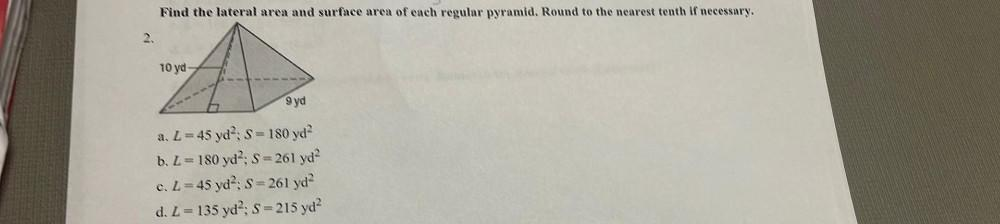Question:

# Find the lateral area and surface area of each regular pyramid. Round to the nearest tenth if necessary. 10 ya 9 yd S a. L=45 ydFind the lateral area and surface area of each regular pyramid. Round to the nearest tenth if necessary. 10 ya 9 yd S a. L=45 yd?; S = 180 yd? b. L = 180 yd : S=261 yd? c. L=45 yd?: S=261 yd? d. L= 135 yd, S=215 yd?